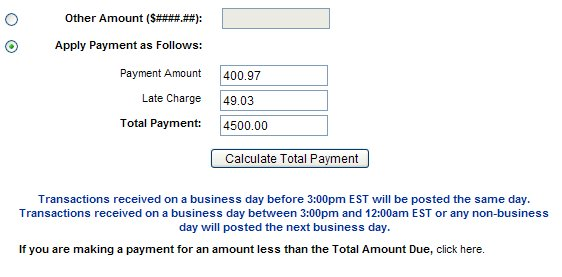"For three years, I've made my car payments on time and in full," Clark S. writes, "and the one time I'm a few days late — whooo boy — do they let me know. Phone calls at home, phone calls at work, letters, emails, you name it. As if that isn't bad enough, then there's the Late Payment Math."Clark continues, "Naturally, the Total Payment amount is read-only and calculated by their system. Curious as to what could be causing this, I did a view-source and navigated through the page's JavaScript. I traced the problem to the following 'clever' JavaScript functions."

```// Javascript doesn't handle decimal numbers very well. So as a
// workaraound, we convert the floating points to real numbers, perform
// the operation and then convert it again back to floating points
function fnCalculateTotalPayment() {
var inputs = null;
var oForm = window.document.SchedulePaymentConfirmationFormBean;

if(document.getElementById('applyPaymentAsFollows') != undefined) {
oForm.totalPayment.value = '';

inputs = document.getElementById('applyPaymentAsFollows')
.getElementsByTagName("input");

if(!validateApplyPaymentAsFollows(inputs)) {
return false;
}

var total = 0;
var charges = 0;
for(var j=0; j<inputs.length; j++) {
if(inputs[j].name != 'totalPayment' && inputs[j].value.trim() != '') {
charges = formatCharges(inputs[j].value);
total += parseInt(charges, 10);
}
}

if(total == 0) {
return false;
}

total = total / 100;

if(parseFloat(total) >= 100000) {
return false;
}

var zeroDecimal = new RegExp(/^\d{0,9}\$/);
//Try out
var singleDecimal = new RegExp(/^\d{0,9}\.\d{1}\$/);

if(zeroDecimal.test(total)) {
total += ".00";
} else if(singleDecimal.test(total)) {
total += "0";
}

oForm.totalPayment.value = total;
}
return true;
}

function formatCharges(charges) {
var singleDecimal = new RegExp(/^\d{0,9}\.\d{2}\$/);
if(singleDecimal.test(charges)) {
charges = charges + "0";
}

var split = charges.split(".");
charges = split + split;

return charges;
}```

"While I admire that they knew enough to use the second argument for parseInt()," Clark adds, "I cannot help but be disgusted by the part where they apparently missed that, in JavaScript, some any number += '0' is basically number * 10."

"I did end up calling customer service, but they had a fairly difficult time understanding that I wanted to help them fix a blatantly obvious error on their website. Not that it mattered: they couldn't refund the late fee, anyway."[Advertisement] BuildMaster allows you to create a self-service release management platform that allows different teams to manage their applications. Explore how!